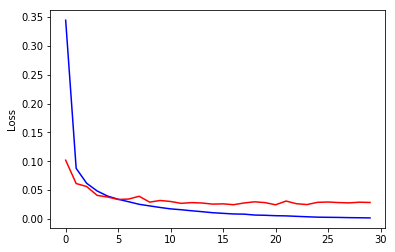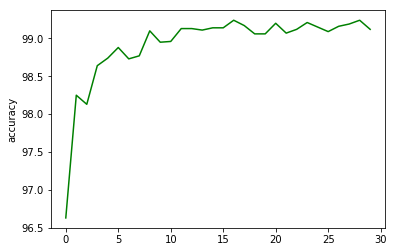# Pytorch Tutorial 001

Lets train a simple CNN on MNIST dataset.

## Defining a simple convolutional neural network

import torch
import torch.nn as nn
import torch.nn.functional as F

class Net(nn.Module):
def __init__(self):
super(Net, self).__init__()
self.conv1 = nn.Conv2d(1, 20, 5, 1)
self.conv2 = nn.Conv2d(20, 50, 5, 1)
self.fc1 = nn.Linear(4*4*50, 500)
self.fc2 = nn.Linear(500, 10)

def forward(self, x):
x = F.relu(self.conv1(x))
x = F.max_pool2d(x, 2, 2)
x = F.relu(self.conv2(x))
x = F.max_pool2d(x, 2, 2)
x = x.view(-1, 4*4*50)
x = F.relu(self.fc1(x))
x = self.fc2(x)
return F.log_softmax(x, dim=1)
net = Net()
print(net)

Net(
(conv1): Conv2d(1, 20, kernel_size=(5, 5), stride=(1, 1))
(conv2): Conv2d(20, 50, kernel_size=(5, 5), stride=(1, 1))
(fc1): Linear(in_features=800, out_features=500, bias=True)
(fc2): Linear(in_features=500, out_features=10, bias=True)
)


## Configuring the network training parameters

The configuration to train the network are as below

train_batch_size=64
test_batch_size=1000
epochs =30
lr=0.01
momentum = 0.5
no_cuda = False
seed = 1
log_interval = 1000
save_model = True


We use two loader for training set and test set. You can also use an optional validation set.

from torchvision import datasets, transforms
use_cuda = False#not no_cuda and torch.cuda.is_available()
torch.manual_seed(seed)

device = torch.device("cuda" if use_cuda else "cpu")

kwargs = {'num_workers': 1, 'pin_memory': True} if use_cuda else {}
transform=transforms.Compose([transforms.ToTensor(),
transforms.Normalize((0.1307,), (0.3081,))])),
batch_size=train_batch_size, shuffle=True, **kwargs)

transforms.ToTensor(),transforms.Normalize((0.1307,), (0.3081,))])),

batch_size=test_batch_size, shuffle=True, **kwargs)


Downloading http://yann.lecun.com/exdb/mnist/train-images-idx3-ubyte.gz
Processing...
Done!


## Setting up the Optimizer to optimize the loss function

Using a simple stochastic gradient descent with nestrov’s momentum

from IPython.display import display, Math, Latex
display(Math(r'w:= w - \eta \sum_{i=1}^{n}\delta Q_{i}(w)/n'))

import torch.optim as optim
model = Net().to(device)
optimizer = optim.SGD(model.parameters(), lr=lr, momentum=momentum)

def train(model, device, train_loader, optimizer, epoch,log_interval):
model.train()
avg_loss = 0
# in training loop:
for batch_idx, (data, target) in enumerate(train_loader):
data, target = data.to(device), target.to(device)
output = model(data)
loss = F.nll_loss(output, target)
loss.backward()
optimizer.step() # Does the update
avg_loss+=F.nll_loss(output, target, reduction='sum').item()

if batch_idx % log_interval == 0:
print('Train Epoch: {} [{}/{} ({:.0f}%)]\tLoss: {:.6f}'.format(
100. * batch_idx / len(train_loader), loss.item()))
return avg_loss

model.eval()
test_loss = 0
correct = 0
data, target = data.to(device), target.to(device)
output = model(data)
test_loss += F.nll_loss(output, target, reduction='sum').item() # sum up batch loss
pred = output.argmax(dim=1, keepdim=True) # get the index of the max log-probability
correct += pred.eq(target.view_as(pred)).sum().item()

print('\nTest set: Average loss: {:.4f}, Accuracy: {}/{} ({:.0f}%)\n'.format(
accuracy = 100. * correct / len(test_loader.dataset)
return test_loss,accuracy

train_losses = []
test_losses = []
accuracy_list = []
for epoch in range(1, epochs + 1):
trn_loss = train(model, device, train_loader, optimizer, epoch,log_interval)
train_losses.append(trn_loss)
test_losses.append(test_loss)
accuracy_list.append(accuracy)

if (save_model):
torch.save(model.state_dict(),"mnist_cnn.pt")

Train Epoch: 1 [0/60000 (0%)]	Loss: 2.300039

Test set: Average loss: 0.1018, Accuracy: 9663/10000 (97%)

Train Epoch: 2 [0/60000 (0%)]	Loss: 0.146140

Test set: Average loss: 0.0614, Accuracy: 9825/10000 (98%)

Train Epoch: 3 [0/60000 (0%)]	Loss: 0.052315

Test set: Average loss: 0.0562, Accuracy: 9813/10000 (98%)

Train Epoch: 4 [0/60000 (0%)]	Loss: 0.019015

Test set: Average loss: 0.0409, Accuracy: 9864/10000 (99%)

Train Epoch: 5 [0/60000 (0%)]	Loss: 0.010967

Test set: Average loss: 0.0380, Accuracy: 9874/10000 (99%)

Train Epoch: 6 [0/60000 (0%)]	Loss: 0.127716

Test set: Average loss: 0.0338, Accuracy: 9888/10000 (99%)

Train Epoch: 7 [0/60000 (0%)]	Loss: 0.027539

Test set: Average loss: 0.0345, Accuracy: 9873/10000 (99%)

Train Epoch: 8 [0/60000 (0%)]	Loss: 0.004211

Test set: Average loss: 0.0394, Accuracy: 9877/10000 (99%)

Train Epoch: 9 [0/60000 (0%)]	Loss: 0.090090

Test set: Average loss: 0.0292, Accuracy: 9910/10000 (99%)

Train Epoch: 10 [0/60000 (0%)]	Loss: 0.125310

Test set: Average loss: 0.0321, Accuracy: 9895/10000 (99%)

Train Epoch: 11 [0/60000 (0%)]	Loss: 0.008019

Test set: Average loss: 0.0303, Accuracy: 9896/10000 (99%)

Train Epoch: 12 [0/60000 (0%)]	Loss: 0.035579

Test set: Average loss: 0.0271, Accuracy: 9913/10000 (99%)

Train Epoch: 13 [0/60000 (0%)]	Loss: 0.018132

Test set: Average loss: 0.0284, Accuracy: 9913/10000 (99%)

Train Epoch: 14 [0/60000 (0%)]	Loss: 0.008078

Test set: Average loss: 0.0276, Accuracy: 9911/10000 (99%)

Train Epoch: 15 [0/60000 (0%)]	Loss: 0.007842

Test set: Average loss: 0.0258, Accuracy: 9914/10000 (99%)

Train Epoch: 16 [0/60000 (0%)]	Loss: 0.001491

Test set: Average loss: 0.0262, Accuracy: 9914/10000 (99%)

Train Epoch: 17 [0/60000 (0%)]	Loss: 0.001637

Test set: Average loss: 0.0248, Accuracy: 9924/10000 (99%)

Train Epoch: 18 [0/60000 (0%)]	Loss: 0.011664

Test set: Average loss: 0.0277, Accuracy: 9917/10000 (99%)

Train Epoch: 19 [0/60000 (0%)]	Loss: 0.023257

Test set: Average loss: 0.0297, Accuracy: 9906/10000 (99%)

Train Epoch: 20 [0/60000 (0%)]	Loss: 0.000937

Test set: Average loss: 0.0284, Accuracy: 9906/10000 (99%)

Train Epoch: 21 [0/60000 (0%)]	Loss: 0.002732

Test set: Average loss: 0.0246, Accuracy: 9920/10000 (99%)

Train Epoch: 22 [0/60000 (0%)]	Loss: 0.000329

Test set: Average loss: 0.0311, Accuracy: 9907/10000 (99%)

Train Epoch: 23 [0/60000 (0%)]	Loss: 0.002051

Test set: Average loss: 0.0264, Accuracy: 9912/10000 (99%)

Train Epoch: 24 [0/60000 (0%)]	Loss: 0.001115

Test set: Average loss: 0.0250, Accuracy: 9921/10000 (99%)

Train Epoch: 25 [0/60000 (0%)]	Loss: 0.001492

Test set: Average loss: 0.0288, Accuracy: 9915/10000 (99%)

Train Epoch: 26 [0/60000 (0%)]	Loss: 0.000727

Test set: Average loss: 0.0294, Accuracy: 9909/10000 (99%)

Train Epoch: 27 [0/60000 (0%)]	Loss: 0.008881

Test set: Average loss: 0.0284, Accuracy: 9916/10000 (99%)

Train Epoch: 28 [0/60000 (0%)]	Loss: 0.003079

Test set: Average loss: 0.0279, Accuracy: 9919/10000 (99%)

Train Epoch: 29 [0/60000 (0%)]	Loss: 0.000659

Test set: Average loss: 0.0291, Accuracy: 9924/10000 (99%)

Train Epoch: 30 [0/60000 (0%)]	Loss: 0.000261

Test set: Average loss: 0.0286, Accuracy: 9912/10000 (99%)


## Plotting the losses and Accuracy

import matplotlib.pyplot as plt
%matplotlib inline
plt.plot(train_losses,'b')
plt.plot(test_losses,'r')
plt.ylabel('Loss')
plt.show()
plt.plot(accuracy_list,'g')
plt.ylabel('accuracy')
plt.show()